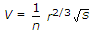# Civil Engineering - Waste Water Engineering

### Exercise :: Waste Water Engineering - Section 7

6.

The formulaused for determining flow velocities in sewers, is known as

 A. Chezy's formula B. Bazin's formula C. Kutter's formula D. Manning's formula.

Explanation:

No answer description available for this question. Let us discuss.

7.

The sewage treatment units in which anaerobic decomposition of organic matter is used, are called

 A. imhoff tanks B. trickling filters C. sludge sedimentation tanks D. none of these.

Explanation:

No answer description available for this question. Let us discuss.

8.

The spacing of man holes along a straight portion of a sewer is 300 m, the diameter of the sewer may be

 A. 0.9 cm B. 1.2 m C. 1.5 m D. > 1.5 m.

Explanation:

No answer description available for this question. Let us discuss.

9.

The most efficient cross section of sewers in a separate sewerage system is

 A. parabolic B. circular C. rectangular D. new egged.

Explanation:

No answer description available for this question. Let us discuss.

10.

If a 2% solution of sewage sample is incubated for 5 days at 20°C and the dissolved oxygen depletion was found to be 8 mg/l. The BOD of the sewage is

 A. 100 mg/l B. 200 mg/l C. 300 mg/l D. 400 mg/l.